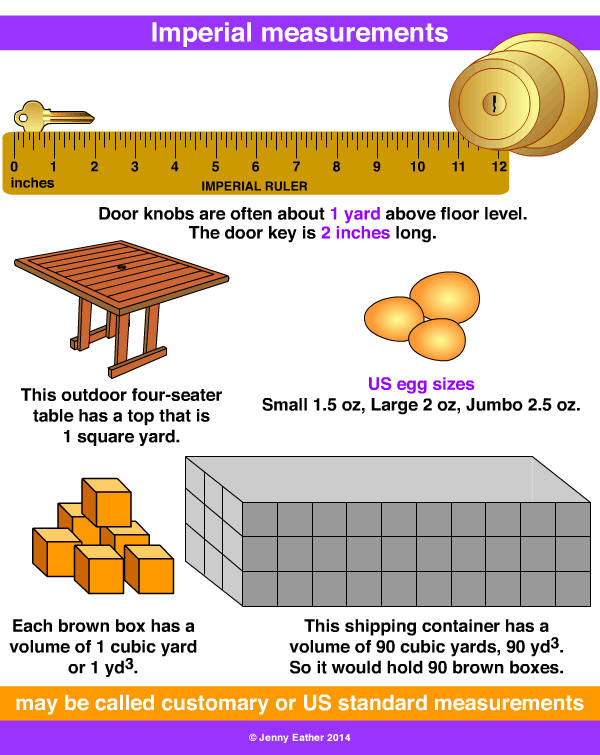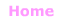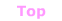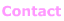Aa Bb Cc Dd Ee Ff Gg Hh Ii Jj Kk Ll Mm Nn Oo Pp Qq Rr Ss Tt Uu Vv Ww Xx Yy Zz

Ii

Imperial system

• the Imperial system of measurement is an old measurement system
based on everyday activities that originated in England.
• the US Standard or customary units developed from this system.
• most countries use the metric system of measurement but
Imperial units may remain in everyday use.

EXAMPLES:Imperial Units ~ Length

12 inches = 1 foot
3 feet = 1 yard
22 yards = 1 chain
10 chains = 1 furlong
8 furlongs = 1 mile
5280 feet = 1 mile
1760 yards = 1 mile

Imperial Units ~ Area

144 square inches = 1 square foot
9 square feet = 1 square yard
4,840 square yards = 1 acre
640 acres = 1 square mile

Imperial Units ~ Volume

1728 cubic inches = 1 cubic foot
27 cubic feet = 1 cubic yard

Imperial Units ~ Fluid Volume

8 fluid ounces = 1 cup (US)
10 fluid ounces = 1 cup (UK)
16 fluid ounces = 1 pint (US)
20 fluid ounces = 1 pint (UK)
2 pints = 1 quart
4 quarts = 1 gallon

1 gallon = 4 quarts, 8 pints, 16 cups

Imperial Units ~ Weight

437.5 grains = 1 ounce
16 ounces = 1 pound
14 pounds = 1 stone
8 stones (112 pounds) = 1 hundredweight (UK)
100 pounds = 1 hundredweight (US)
20 hundredweight = 1 ton
1 ton = 2240 pounds (UK)
1 ton = 2000 pounds (US)© Jenny Eather 2014. All rights reserved.